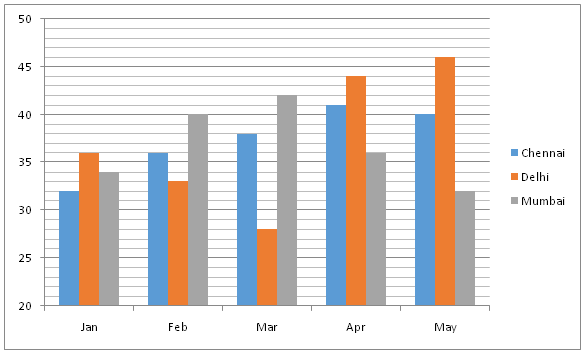# SBI Clerk Prelims 2021 Quantitative Aptitude Questions (Day-49)

Dear Aspirants, Our IBPS Guide team is providing new series of Quantitative Aptitude Questions for SBI Clerk Prelims 2020 so the aspirants can practice it on a daily basis. These questions are framed by our skilled experts after understanding your needs thoroughly. Aspirants can practice these new series questions daily to familiarize with the exact exam pattern and make your preparation effective.

Start Quiz

Bar graph

Directions (01-05): Study the following information carefully and answer the questions given below.

The following bar graph shows the average temperature (In 0C) in 3 different cities in 5 different months.1) Find the ratio between the temperature of Chennai and Mumbai together in the month of Jan to that of Apr.

A.6: 7

B.8: 11

C.12: 19

D.15: 23

E.None of these

2) Find the difference between the temperature of all the given cities in the month of Feb and that of May.

A.150 0C

B.90 0C

C.270 0C

D.340 0C

E.None of these

3) The temperature of Chennai in all the given months together is approximately what percentage of the temperature of Mumbai in all the given months together?

A.120 %

B.94 %

C.86 %

D.102 %

E.77 %

4) The temperature of all the given cities in the month of Jan is approximately what percentage more/less than the temperature of all the given cities in the month of Mar?

A.6 % less

B.15 % more

C.15 % less

D.6 % more

E.24 % less

5) Find the difference between the temperature of Delhi and that of Mumbai in all the given months together.

A.180 0C

B.70 0C

C.30 0C

D.110 0C

E.None of these

Simplification

Directions (06-10): What value should come in the place of (?) in the following questions?

6) 4785.23 + 25 × 1.25 + 38383.33 =? + 25123.12

A.17076.69

B.18076.69

C.18876.69

D.17976.79

E.None of these

7) 285 % of 1560 + 25 % of 4096 =? – 152 * 19

A.8358

B.8458

C.7358

D.7458

E.8258

8)
13824 × 75 % of 392 – √5184 × 20 % of 225 =?

A.4624

B.3816

C.5016

D.5024

E.4724

9) 65 % of 35 % of 48 % of 22500 =? – 2/7 × 12061

A.5993

B.5903

C.6993

D.6903

E.None

10) (642.3 × 168.1 × 2560.2)/( 164.3 ÷ 411.2) = 412.1 × 2?

A.24.8

B.25.8

C.26.8

D.23.8

E.28.8

The temperature of Chennai and Mumbai together in the month of Jan

= >32 + 34 = 66 0C

The temperature of Chennai and Mumbai together in the month of Apr

= > 41 + 36 = 77 0C

Required ratio = 66: 77 = 6: 7

The temperature of all the given cities in the month of Feb

= > 36 + 33 + 40 = 109 0C

The temperature of all the given cities in the month of May

= >40 + 46 + 32 = 118 0C

Required difference = 118 – 109 = 90 C

The temperature of Chennai in all the given months together

= >(32 + 36 + 38 + 41 + 40) = 1870 C

The temperature of Mumbai in all the given months together

= >(34 + 40 + 42 + 36 + 32) = 1840 C

Required % = (187 / 184) * 100 = 101.63 % = 102 %

The temperature of all the given cities in the month of Jan

= >32 + 36 + 34 = 1020 C

The temperature of all the given cities in the month of Mar

= >38 + 28 + 42 = 1080 C

Required % = [(108 – 102) / 108] * 100 = 5.55 % = 6 % less

The temperature of Delhi in all the given months together

= >(36 + 33 + 28 + 44 + 46) = 1870 C

The temperatures of Mumbai in all the given months together

= >(34 + 40 + 42 + 36 + 32) = 1840 C

Required difference = 187 – 184 = 30 C

4785.23 + 25 × 1.25 + 38383.33 = ? + 25123.12

4785.23 + 31.25 + 38383.33 = ? + 25123.14

43199.81 – 25123.14 = ?

18076.69 = ?

285/100 × 1560 + 25/100 × 4096 =? – 152 * 19

4446 + 1024 = ? – 2888

8358 =?

∛13824 × 75/100 × 392 – √5184 × 20/100 × 225 =?

24 × 294 – 72 × 45 =?

7056 – 3240 =?

?= 3816

65/100 × 35/100 × 48/100 × 22500 = ? – 2/7 × 12061

2457 + 3446 =?

5903= ?

(26(2.3) × 24(8.1) × 28(0.2))/( 24(4.3) ÷ 22(11.2)) = 22(12.1) × 2?

2(13.8 + 32.4 + 1.6) – (17.2 – 22.4) = 224.2 × ?

? = 28.8

 Check Here to View SBI Clerk Prelims 2021 Quantitative Aptitude Questions Day – 48 Day – 47 Day – 46